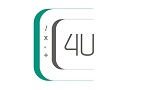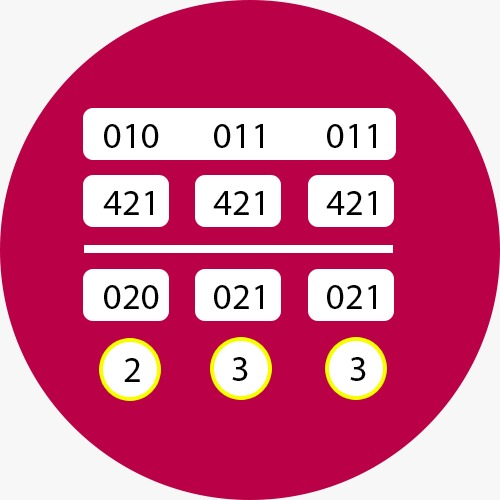﻿ Binary to Octal Converter | Binary to Octal Conversion# Binary to Octal Converter

Use our Binary to Octal converter a free online calculator / conversion tool to convert binary value into octal number system. The octal numeral system, or oct for short, is the base-8 number system, and uses the digits 0 to 7. Octal numerals can be made from binary numerals by grouping consecutive binary digits into groups of three (starting from the right)### Standard Number System conversion table with values for first 15 numbers in each format

DecimalBinaryOctalHexadecimal
0000000
1000111
2001022
3001133
4010044
5010155
6011066
7011177
81000108
91001119
10101012A
11101113B
12110014C
13110115D
14111016E
15111117F

### Binary Number System

A Binary number system has only two digits that are 0 and 1. Every number (value) represents with 0 and 1 in this number system. The base of binary number system is 2, because it has only two digits.

### Octal Number System

Octal number system has only eight (8) digits from 0 to 7. Every number (value) represents with 0,1,2,3,4,5,6 and 7 in this number system. The base of octal number system is 8, because it has only 8 digits.

#### How to Convert Binary to Octal Values

An easy way to convert from binary to octal is to group binary digits into sets of three, starting with the least significant (rightmost) digits.

Example

Let's convert the binary value 11100101 into octal number system

First group binary digits into sets of three, starting with the least significant (right most) digits. We get: 11 100 101

Now pad the most significant digits with zeros if necessary to complete a group of three. We get: 011 100 101

Then, look up each group in a number system table, We get: 011 -> 3; 100 -> 4; 101 -> 5

Result: The binary to octal conversion of 11100101 is 345

### Applications and Advantages of Octal Number Systems

The main advantage of using Octal numbers is that it uses less digits than decimal and Hexadecimal number system. Octal became widely used in computing when systems such as the UNIVAC 1050, PDP-8, ICL 1900 and IBM mainframes employed 6-bit, 12-bit, 24-bit or 36-bit words. Octal was an ideal abbreviation of binary for these machines because their word size is divisible by three (each octal digit represents three binary digits).